### Dependent Types and Multi-monadic Effects in F*

Nikhil Swamy, Cătălin Hriţcu,
C. Keller, A. Rastogi, G. Martínez, G. Plotkin, J. Protzenko,
A. Delignat-Lavaud, K. Bhargavan, C. Fournet,
M. Kohlweiss, J-K. Zinzindohoue, S. Zanella-Beguelin,
A. Anand, J. Chen, S. Forest, P-Y. Strub,

Microsoft Research, INRIA Paris, U Maryland, U Edinburgh,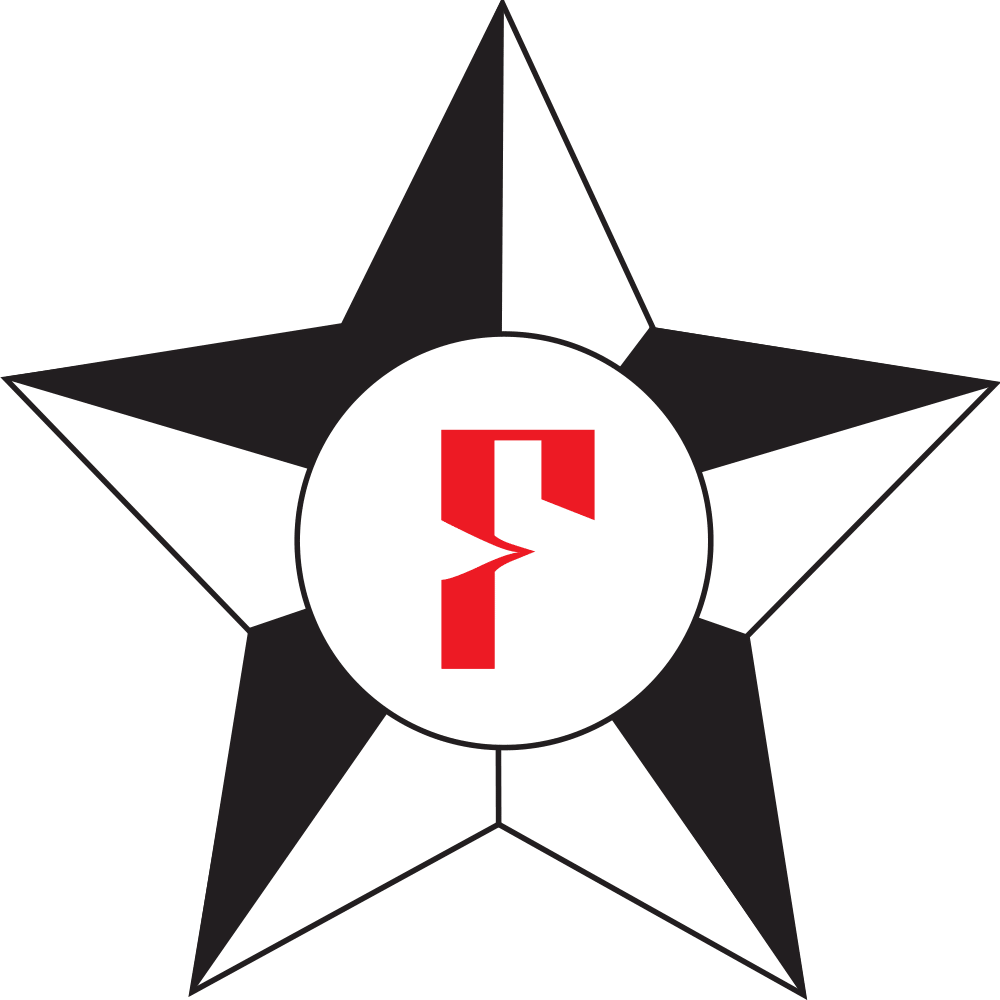### Shall the twain ever meet?

The two, mostly disjoint sides of program verification research

Interactive proof assistants Semi-automated verifiers of imperative programs
Coq, CompCert,   air Dafny, Verve,
Agda, Bedrock, Why3, miTLS
Lean, 4 colors   gap VCC
• In the left corner:
Expressive logics, but only purely functional programming

• In the right:
Only first-order logic, but rich, effectful programming

### Bridging the gap: F*

• Functional programming language with effects

• predictable cost model, type and effect inference
• like OCaml, F#, Standard ML, Haskell, ..
• Semi-automated verification system using SMT

• like Dafny, FramaC, Why3, VCC,
• Interactive proof assistant based on dependent types

• full dependency, inductive types, universe polymorphism
• like Coq, Lean, Agda,
• Also in the gap: ATS, HTT, Idris, Trellys,

### Illustrating the threefold nature of F*

• F* is programmed in F* and bootstraps in OCaml or F#

• We use it daily for general-purpose programming
• Verified implementation of upcoming TLS 1.3 protocol

• Relying on SMT for verifying effectful programs
•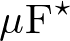is (partially) formalized in F*

• We use it as a proof assistant for PL metatheory

### A first example: Quicksort

let rec quicksort f = function
| [] -> []
| pivot::tl -> let hi, lo = partition (f pivot) tl in
quicksort f lo @ pivot::quicksort f hi

• Total correctness of quicksort, proven via a combination of F* type-checking and SMT solving

  val quicksort: f:total_order a
-> l:list a
-> Tot (m:(list a){sorted f m /\ forall i. count i l = count i m})
(decreases (length l)) 

### Semantic termination checking

val quicksort: f:total_order a
-> l:list a
-> Tot //Total function: semantic termination checking
(m:(list a){sorted f m /\ forall i. count i l = count i m})
(decreases (length l)) //termination metric
• From library:

let rec length = function [] -> 0 | _::tl -> 1 + length l

### Full dependency and refinement types

val quicksort: f:total_order a
-> l:list a
-> Tot (m:(list a){sorted f m /\ forall i. count i l = count i m})
(* ^^^^^^^^^^^refinement types^^^^^^^^^^^^^^^ *)
• From library:

let rec sorted f = function
| [] | [_] -> true
| x::y::tl -> f x y && sorted f (y::tl)

let rec count i = function
| [] -> 0
| hd::tl when i=hd -> 1 + count tl
| _::tl -> count tl

### Refinements at higher order

val quicksort: f:total_order a //refinements at higher order
-> l:list a
-> Tot (m:list a{sorted f m /\ forall i. count i l = count i m})
• From library:

type total_order a = f:(a -> a -> Tot bool){
(forall a. f a a)                                           (* reflexivity   *)
/\ (forall a1 a2. f a1 a2 /\ f a2 a1  ==> a1 = a2)          (* anti-symmetry *)
/\ (forall a1 a2 a3. f a1 a2 /\ f a2 a3 ==> f a1 a3)        (* transitivity  *)
/\ (forall a1 a2. f a1 a2 \/ f a2 a1)                       (* totality  *)
}

### Doesn't sound like the F* you've heard about before?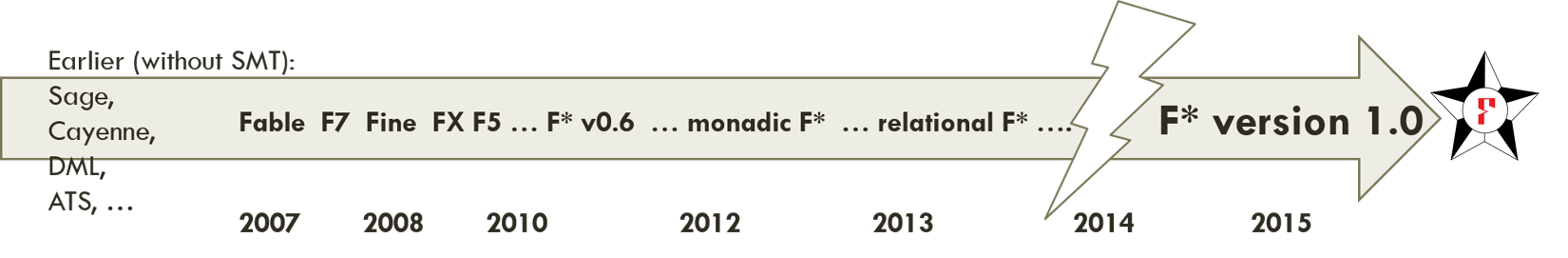• Open source and cross-platform

• Full dependent types, inductives, universe polymorphism

• Type and effect inference (but no invariant inference)

• SMT automation for large boring proofs

• Programs as proof terms, when SMT fails

• Semantic termination checking

• Effects specified using a lattice of predicate-transformer monads (Dijkstra monads) – rest of the talk

### Programming with effects, like in ML

• Divergence:

let server () = let req = get () in ...; server ()
• Exceptions:

let rec find f = function
| [] -> raise Not_found
| hd::tl -> if f hd then hd else find f tl
• State:

let ctr =
let i = ref 0 in
fun () -> incr i; !i

### Indexed computation types track effects

• Unconditionally pure: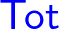1 + 1       : Tot int  
• Conditionally pure: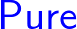factorial x : Pure int (requires (x >= 0)) (ensures (fun y -> y >= 0)) 
• By subtyping:

type nat = x:int{x >= 0}
factorial 1 : Tot nat
• Stateful: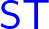x := 17     : ST unit (requires (fun h' -> h' contains x))
(ensures (fun h' _ h -> h = upd h' x 17))

### Effect inclusion via a user-defined lattice

• Effect inclusion:is lifted to1 + 1       : ST int (requires (fun h' -> True))
(ensures (fun h' x h -> x=1+1 /\ h=h'))
• Effects ordered in a lattice, for instance: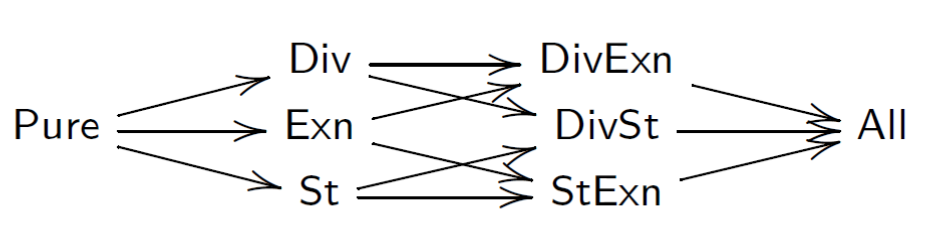Can also pick other effects (e.g., Ghost, IO, NonDet, )

• Each point in the lattice is a monad of predicate transformers (aka a Dijkstra monad)

•.wp: a monad for pure computations at the bottom

Pure.post a = a -> Type
Pure.pre    = Type
Pure.wp a   = Pure.post a -> Pure.pre
Pure.return (x:a) : Pure.wp a  = fun post -> post x
Pure.bind (wp1:Pure.wp a) (wp2: a -> Pure.wp b) : Pure.wp b =
fun post -> wp1 (fun x -> wp2 x post)
•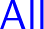.wp: state, exceptions, and divergence monad

All.post a = mem -> (a + exn) -> Type
All.pre    = mem -> Type
All.wp a   = All.post a -> All.pre
All.return (x:a) : All.wp a  = fun post mem -> post mem (Inl x)
All.bind (wp1:All.wp a) (wp2:a -> All.wp b) : All.wp b =
fun post m0 -> wp1 (fun m1 -> function
| Inl x  -> wp2 x post m1
| Inr ex -> post m1 (Inr ex)) m0

###.wp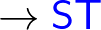.wp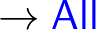.wp

ST.post a = mem -> a -> Type
ST.pre    = mem -> Type
ST.wp   a = ST.post a -> ST.pre
ST.return x = fun post m0 -> post m0 x
ST.bind wp1 wp2 = fun post m0 -> wp1 (fun m1 x -> wp2 x m1 post) m0
• Lifts witnessing effect inclusion

lift_pure_st : Pure.wp a -> ST.wp a = fun wp post m0 -> wp (post m0)
lift_st_all  : ST.wp a -> All.wp a = fun wp post -> wp (fun m1 x -> post m1 (Inl x))
• Lifts must commute (monad morphism laws)

    All.bind (lift_st_all wpa) (lift_st_all . wp') =ext= lift_st_all (ST.bind wp wp')

### Benefits of our multi-monadic approach

• Flexibility in choosing and structuring the effects

• Generic WP calculus that works for all effects

• type inference automatically lifts effects as needed
• VC complexity reflects only effects that are actually used

• e.g., no exponentially many paths due to exceptions in code that is syntactically exception-free

• Automatically derive Dijkstra monads from expression-level monads, via continuation-passing style transformation

(mem -> mem × a)* = mem -> (mem × a -> Type) -> Type
= (mem -> a -> Type) -> (mem -> Type)
(* ^^^^^^^^^^^^^^^^      ^^^^^^^^^^^   *)
(*  post-condition   ->  pre-condition *)
• any effect, not just those from ML (e.g. Wysteria SMPC)

• reify and reflect between primitive and encoded effects

• enables extrinsic reasoning about effectful code

• relational reasoning, forgetting effects, fictional purity,

### Ongoing and future work

• Verified implementation of upcoming TLS 1.3 protocol

• Developing F* further as a proof assistant:

• plans for adding Mtac-inspired tactic language
• Extending metatheory, towards self-certification

• e.g. formalizing encoding from F* to SMT
• Making F* more usable (e.g. better error messages)

### Conclusion

• Many semi-automated verifiers for first-order languages

• Relatively simple logics, effectful, good SMT automation
• Many interactive proof assistants with dependent types

• Good control, but automation lacking; effects via encoding
• F* tries to span the divide